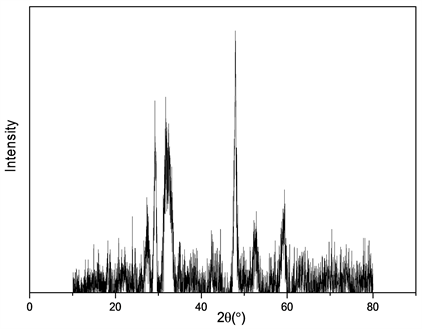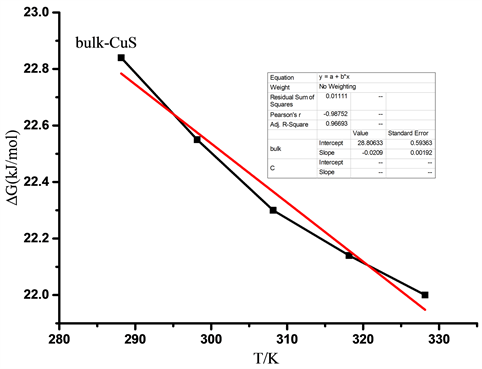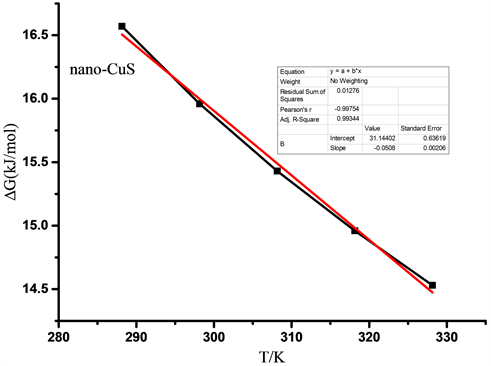﻿ 溶解度法研究硫化铜表面热力学性质

# 溶解度法研究硫化铜表面热力学性质Study on Thermodynamic Properties of Copper Sulfide Surface by Solubility Method

Abstract: Nanocrystalline copper sulphide particles were prepared by coprecipitation method. The solution equilibrium constants of nanometer copper sulfide and corresponding bulk copper sulfide at 288.15 K, 298.15 K, 308.15 K, 318.15 K, 328.15 K were determined by solubility method. Based on the theory of dissolution thermodynamics, the dissolution thermodynamic function of copper sulfide was further calculated, and the surface thermodynamic function of copper sulfide was obtained by comparison with bulk.

1. 引言

2. 实验部分

2.1. 试剂与仪器

2.2. 制备纳米CuS

2.3. 制备块体CuS

2.4. 电导率的测定

3. 结果与讨论

3.1. 溶解热力学函数

$\text{CuS}⇌{\text{Cu}}^{\text{2}+}+{\text{S}}^{\text{2}-}$

${k}_{sp}=c\left({\text{Cu}}^{2+}\right)\cdot c\left({\text{S}}^{2-}\right)={c}^{2}$ (1)(a) 立方体Cu2O的SEM图(b) 立方体Cu2O的XRD谱

Figure 1. SEM images and XRD spectrum of CuS

${\Lambda }_{m}=k/c$ (2)

c为饱和溶液中微溶盐的溶解度，即：

${\Lambda }_{m}\left(盐\right)=k\left(盐\right)/c$ (3)

${\Lambda }_{m}^{\infty }\left(\text{CuS}\right)={\Lambda }_{m}^{\infty }\left({\text{Cu}}^{\text{2+}}\right)+{\Lambda }_{m}^{\infty }\left({\text{S}}^{2-}\right)$ (4)

${\Lambda }_{m}^{\infty }\left({\text{Cu}}^{2+}\right)=5.32×{10}^{-3}\left(\text{S}\cdot {\text{m}}^{\text{2}}\cdot {\text{mol}}^{-\text{1}}\right)×\text{2}=\text{1}0.\text{64}×\text{1}{0}^{-\text{3}}\left(\text{S}\cdot {\text{m}}^{\text{2}}\cdot {\text{mol}}^{-\text{1}}\right)$

${\Lambda }_{m}^{\infty }\left({\text{S}}^{2-}\right)=51.2×{10}^{-3}\left(\text{S}\cdot {\text{m}}^{\text{2}}\cdot {\text{mol}}^{-\text{1}}\right)×\text{2}=\text{1}0\text{2}.\text{41}{0}^{-\text{3}}\left(\text{S}\cdot {\text{m}}^{\text{2}}\cdot {\text{mol}}^{-\text{1}}\right)$

${\Lambda }_{m}^{\infty }\left(\text{CuS}\right)=113.04×\text{1}{0}^{-\text{3}}\left(\text{S}\cdot {\text{m}}^{\text{2}}\cdot {\text{mol}}^{-\text{1}}\right)$

${\Lambda }_{m}\left(\text{CuS}\right)={\Lambda }_{m}^{\infty }\left(\text{CuS}\right)=113.04×{10}^{-3}\left(\text{S}\cdot {\text{m}}^{\text{2}}\cdot {\text{mol}}^{-\text{1}}\right)$

${c}_{{\text{S}}^{2-}}={c}_{\text{CuS}}$

${c}_{\text{CuS}}=\frac{{k}_{\text{CuS}}}{{\Lambda }_{m\left(\text{CuS}\right)}}$ (4)

k (溶液) = k (水) + k (盐)，k (盐) = k (溶液) − k (水)，式中k (盐)是纯微溶盐的电导率。

$C=\frac{{K}_{\text{CuS}}}{\Lambda {\text{m}}_{\text{CuS}}}$

${K}_{sp}={C}^{\text{2}}$

$\Delta {G}_{m}^{\theta }=-RT\text{ln}{K}^{\theta }$Table 1. Conductivity of pure water and copper sulfide solution at different temperaturesTable 2. Conductivity of copper sulfide at different temperaturesTable 3. Concentration of copper sulfide at different temperatures

$\Delta {G}_{m}^{\theta }=-RT\text{ln}{K}^{\theta }$

$\Delta {G}_{m}^{\theta }=\Delta {H}_{m}^{\theta }-T\Delta {S}_{m}^{\theta }$

$\Delta {G}_{m}^{\theta }$ 对温度T作图，并进行线性拟合，得到的直线截距即为溶液标准溶解焓 $\Delta {H}_{m}^{\theta }$ ，单位为KJ∙mol−1；直线斜率的相反数即为纳米颗粒的标准溶解熵 $\Delta {S}_{m}^{\theta }$ ，单位为J∙mol−1∙K−1

3.2. 表面热力学函数Table 4. Dissolution equilibrium constants of copper sulfide at the same temperatureTable 5. Gibbs free energy of copper sulfide at different temperaturesTable 6. Nano-CuS surface thermodynamic functionsFigure 2. The standard dissolved Gibss free energy of bulk copper sulfide varies with temperatureFigure 3. The standard dissolved Gibss free energy of nano-copper sulphide varying with temperature

4. 结论

NOTES

*通讯作者。

 Yu, X.L., Cao, C.B., Zhu, H.S., et al. (2010) Nanometer-Sized Copper Sulfide Hollow Spheres with Strong Optical-Limiting Proper-ties. Advanced Functional Materials, 17, 1397-1401.

 Liu, X.L., Zhu, Y.J., Yang, B., et al. (2012) CuS Nanotubes: Microwave-Solvothermal Synthesis and Photocatalytic Property. Advanced Materials Research, 554-556, 605-609.

 Chung, J.S. and Sohn, H.J. (2002) Electrochemical Behaviors of CuS as a Cathode Material for Lithium Secondary Batteries. Journal of Power Sources, 108, 226-231.
https://doi.org/10.1016/S0378-7753(02)00024-1

 Liu, X., Li, B., Fu, F., et al. (2014) Facile Synthesis of Biocompatible Cysteine-Coated CuS Nanoparticles with High Photothermal Conversion Efficiency for Cancer Therapy. Dalton Transactions, 43, 11709-11715.
https://doi.org/10.1039/C4DT00424H

 Huse, N.P., Dive, A.S. Gattu, K.P., et al. (2017) An Experimental and Theoretical Study on Soft Chemically Grown CuS Thin Film for Photosensor Application. Materials Science in Semiconductor Processing, 67, 62-68.
https://doi.org/10.1016/j.mssp.2017.05.010

 He, J., Ai, L., Liu, X., et al. (2018) Plasmonic CuS Nanodisk Assemblies Based Composite Nanocapsules for NIR-Laser-Driven Synergistic Chemo-Photothermal Cancer Therapy. Journal of Materials Chemistry B, 6, 1035-1043.
https://doi.org/10.1039/C7TB02772A

 Han, S.K., Gu, C., Zhao, S., et al. (2016) Precursor Triggering Synthesis of Self-Coupled Sulfide Polymorphs with Enhanced Photoelectrochemical Properties. Journal of the American Chemical Society, 138, 12913-12919.
https://doi.org/10.1021/jacs.6b06609

 Wang, Q., An, N., Bai, Y., et al. (2013) High Photocatalytic Hydrogen Production from Methanol Aqueous Solution Using the Photocatalysts CuS/TiO2. International Journal of Hydrogen Energy, 38, 10739-10745.
https://doi.org/10.1016/j.ijhydene.2013.02.131

 Lin, R., Huang, P., Ségalini, J., et al. (2009) Solvent Effect on the Ion Ad-sorption from Ionic Liquid Electrolyte into Sub-Nanometer Carbon Pores. Electrochimica Acta, 54, 7025-7032.
https://doi.org/10.1016/j.electacta.2009.07.015

 Li, X., Wan, T., Qiu, J., et al. (2017) In-Situ, Photocalorimetry-Fluorescence Spectroscopy Studies of RhBphotocatalysis over Z-Scheme g-C3N4@Ag@Ag3PO4, Nanocomposites: A Pseudo-Zero-Order Rather Than a First-Order Process. Applied Catalysis B Environmental, 15, 591-602.

 Khairy, M., Ayoub, H.A. and Banks, C.E. (2018) Non-Enzymatic Electrochemical Platform for Parathion Pesticide Sensing Based on Nanometer-Sized Nickel Oxide Modified Screen-Printed Electrodes. Food Chemistry, 255, 104-111.
https://doi.org/10.1016/j.foodchem.2018.02.004

 Xiang, M. (2015) Young Measure Solutions for a Fourth-Order Wave Equation with Variable Growth. Boundary Value Problems, 2015, 123.
https://doi.org/10.1186/s13661-015-0386-5

 Navrotsky, A. (1977) Progress and New Directions in High Temperature Calo-rimetry. Physics & Chemistry of Minerals, 2, 89-104.
https://doi.org/10.1007/BF00307526

 王路得, 黄在银, 范高超, 等. 电化学方法测定纳米材料的热力学函数[J]. 中国科学:化学, 2012, 42(1): 47-51.

 刘晓林, 王路得, 黄在银, 等. 纳米氧化锌热力学函数的微量热法及电化学法测量[J]. 高等学校化学学报, 2015, 36(3): 539-543.

 肖明, 黄在银, 汤焕丰, 等. Ag3PO4表面热力学性质及光催化原位过程热动力学的晶面效应[J]. 物理化学学报, 2017, 33(2): 399-406.

Top